• Last Updated : 19 Feb, 2021

Python is a great language for doing data analysis, primarily because of the fantastic ecosystem of data-centric Python packages. Pandas is one of those packages and makes importing and analyzing data much easier.

`Dataframe.add()` method is used for addition of dataframe and other, element-wise (binary operator add). Equivalent to dataframe + other, but with support to substitute a fill_value for missing data in one of the inputs.

Attention geek! Strengthen your foundations with the Python Programming Foundation Course and learn the basics.

To begin with, your interview preparations Enhance your Data Structures concepts with the Python DS Course. And to begin with your Machine Learning Journey, join the Machine Learning - Basic Level Course

Parameters:

other :Series, DataFrame, or constant
axis :{0, 1, ‘index’, ‘columns’} For Series input, axis to match Series index on
fill_value : [None or float value, default None] Fill missing (NaN) values with this value. If both DataFrame locations are missing, the result will be missing.
level : [int or name] Broadcast across a level, matching Index values on the passed MultiIndex level

Returns: result DataFrame

 `# Importing Pandas as pd``import` `pandas as pd`` ` `# Importing numpy as np``import` `numpy as np`` ` `# Creating a dataframe``# Setting the seed value to re-generate the result.``np.random.seed(``25``)`` ` `df ``=` `pd.DataFrame(np.random.rand(``10``, ``3``), columns ``=``[``'A'``, ``'B'``, ``'C'``])`` ` `# np.random.rand(10, 3) has generated a``# random 2-Dimensional array of shape 10 * 3``# which is then converted to a dataframe`` ` `df`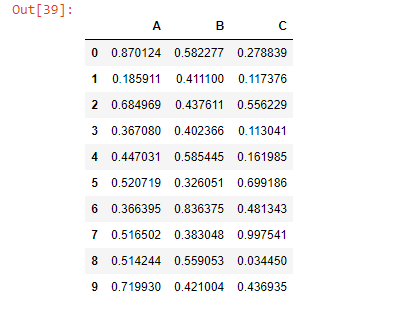Note: `add() `function is similar to ‘+’ operation but, `add()` provides additional support for missing values in one of the inputs.

 `# We want NaN values in dataframe. ``# so let's fill the last row with NaN value``df.iloc[``-``1``] ``=` `np.nan`` ` `df`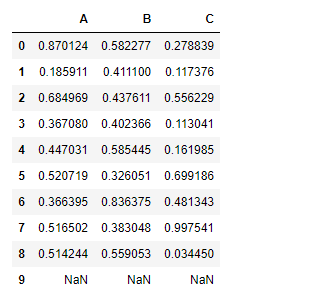Adding a constant value to the dataframe using `add()` function:

 `# add 1 to all the elements``# of the data frame``df.add(``1``)`` `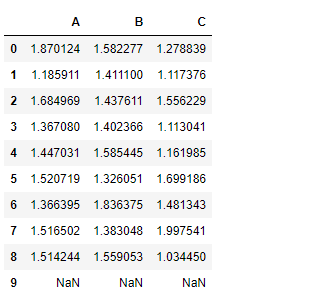Notice the output above, no addition took place for the nan cells in the df `dataframe.add() `function has an attribute `fill_value`. This will fill the missing value(Nan) with the assigned value. If both dataframe values are missing then, the result will be missing.

Let’s see how to do it.

 `# We have given a default value``# of '10' for all the nan cells``df.add(``1``, fill_value ``=` `10``)`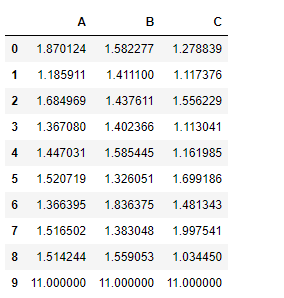All the nan cells has been filled with 10 first and then 1 is added to it.

For Series input, the dimension of the indexes must match for both data frame and series.

 `# Create a Series of 10 values``tk ``=` `pd.Series(np.ones(``10``))`` ` `# tk is a Series of 10 elements``# all filled with 1`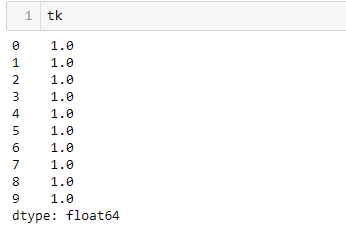`# Add tk(series) to the df(dataframe)``# along the index axis``df.add(tk, axis ``=``'index'``)`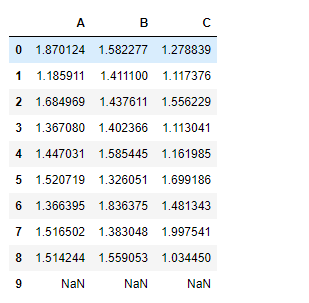Adding one data frame with other data frame

 `# Create a second dataframe``# First set the seed to regenerate the result``np.random.seed(``10``)`` ` `# Create a 5 * 5 dataframe``df2 ``=` `pd.DataFrame(np.random.rand(``5``, ``5``), columns ``=``[``'A'``, ``'B'``, ``'C'``, ``'D'``, ``'E'``])`` ` `df2`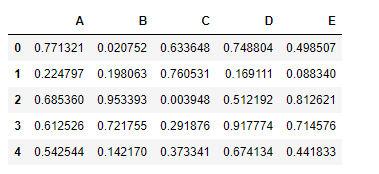Let’s perform element-wise addition of these two data frames

 `df.add(df2)`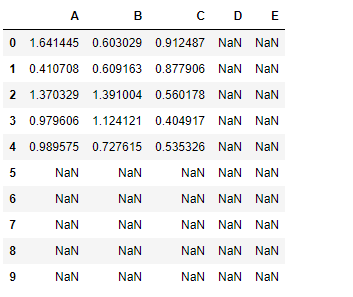Notice the resulting dataframe has dimension 10*5 and it has nan value in all those cells for which either of the dataframe has nan value.

Let’s fix it –

 `# Set a default value of 10 for nan cells``# nan value won't be filled for those cells``# in which both data frames has nan value``df.add(df2, fill_value ``=` `10``)`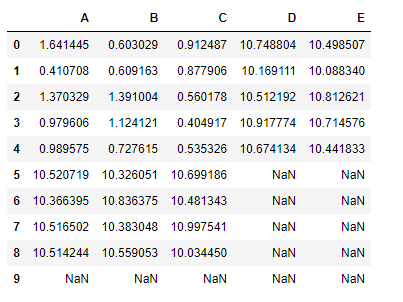My Personal Notes arrow_drop_up